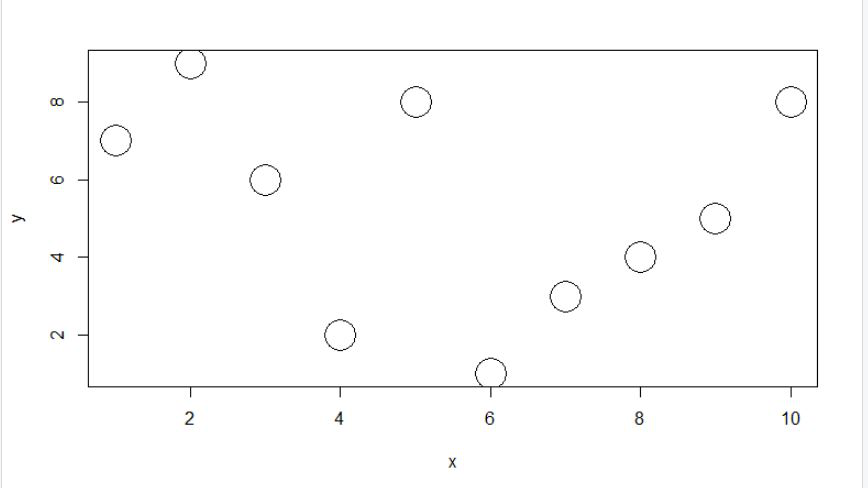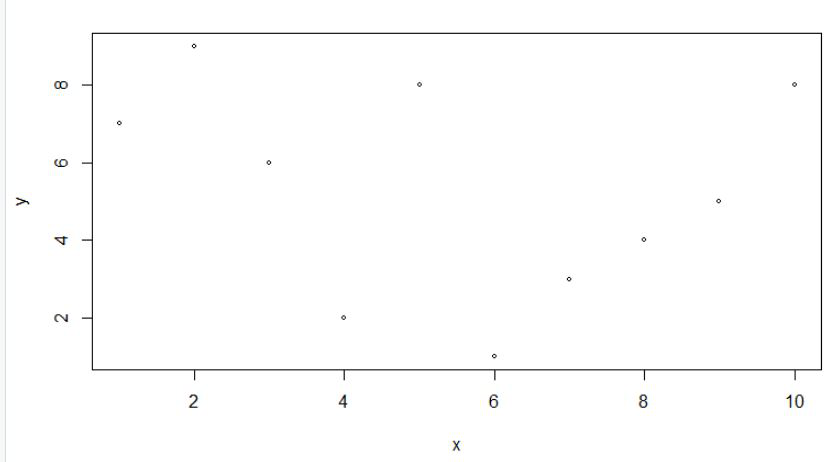Related Articles

# Control the Size of the Points in a Scatterplot in R

• Last Updated : 23 May, 2021

In this article, we are going to see how to control the size of the points in a scatterplot in R Programming language.

We will Control the size of the points in a scatterplot using cex argument of the plot function. In this approach to control the size of the points in a scatterplot, the user needs to call the in-built function plot() and using the cex parameter which will take input value as a float in this function of control the size of the points of the given scatterplot in r language.

`Syntax: plot( Data_x, Data_y, cex)`

Example 1: Increase the point size in a scatterplot.

In this function, we will be increasing the size of the points of the given scatterplot using the cex argument of the plot function of r language. Here cex will be set to 4 which will increase the size of the points of the scatterplot.

## Python3

 `x ``=` `c(``1``, ``2``, ``3``, ``4``, ``5``, ``6``, ``7``, ``8``, ``9``, ``10``)``y ``=` `c(``7``, ``9``, ``6``, ``2``, ``8``, ``1``, ``3``, ``4``, ``5``, ``8``)``   ` `plot(x, y, cex ``=` `4``) `

Output:Example 2: Decreasing the point size in a scatterplot.

In this function, we will be decreasing the size of the points of the given scatterplot using the cex argument of the plot function of r language. Here cex will be set to 0.6 which will decrease the size of the points of the scatterplot.

## R

 `x = ``c``(1, 2, 3, 4, 5, 6, 7, 8, 9, 10)``y = ``c``(7, 9, 6, 2, 8, 1, 3, 4, 5, 8)`` ` `plot``( x, y, cex = 0.6) `

Output:My Personal Notes arrow_drop_up